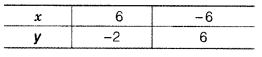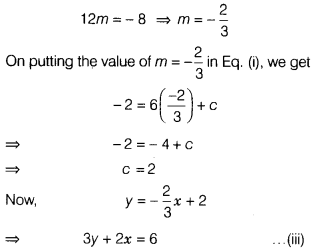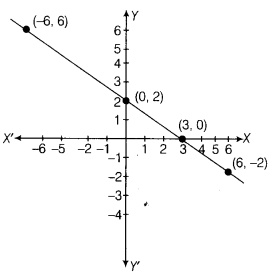# The following observed values of x and y are thought to satisfy a linear equation

The following observed values of x and y are thought to satisfy a linear equation. Write the linear equation.Draw the graph, using the values x and y as given in the above table. At what points, the graph of the linear equation
(i) cuts the X-axis?
(ii) cuts the Y-axis?

Let the linear equation y = mx + c satisfies the points (6-2)and (-6,6).
∴ - 2 = 6 m + c…(i)
and 6 = - 6 m + c…(ii)
On subtracting Eq. (ii) from Eq. (i), we getwhich is the required linear equation and its graph isOn putting y=0 in eq.(iii), we get
0 + 2x = 6
=> x = 3
∴(3, 0) is a solution of the equation.
Again, putting x = 0 in Eq. (iii), we get
3y + 0 = 6
=> y=2
∴(0,2) is a solution of the equation.
(i) The graph of the linear equation will cut X-axis at
(3,0).
(ii) The graph of the linear equation will cut Y-axis at
(0,2).